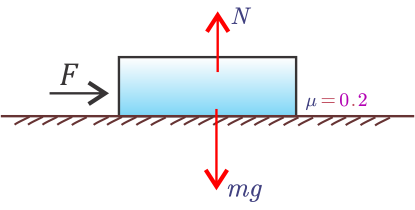Home » Physics » Force of friction equation (friction formula)

# Force of friction equation (friction formula)

In this article learn about force of friction equation (or friction formula). This friction formula is very important while solving problems related to Newton's laws of motion. You may also like to go to class 11 physics notes for more notes and study materials.

Force of friction is a force which acts between two surfaces in contact and when one surface slides on the other surface.
Friction arises due to roughness of two surfaces in contact. Frictional force is a useful force. We are able to walk, drive cars, stop moving cars by applying breaks due to the application of force of friction. You can find lots of application of this force in your day to day life. Frictional forces are not always desirable. For example a lot of energy is wasted in overcoming friction in machines. we can reduce friction between two surfaces through lubrication and polishing. Ball bearing between the two surfaces can also reduce friction between two surfaces. Here sliding friction is converted into rolling friction. Rolling friction is much less then sliding friction
Friction does not depends on the surface areas of objects or bodies under contact. It depends on how hard two objects are pressing against each other and the types of surfaces.
There are two types of frictional forces namely,
1. Static friction
2. Kinetic friction
Frictional force may exist between two surfaces even when there is no relative motion between them. When frictional force comes into action it can cause energy to be dissipated or lost in the form of heat.
Coefficient of friction is the ratio of limiting friction acting on a body to the normal reaction acting on it. It is denoted by Greek letter $\mu$. Coefficient of friction in physical sense describe how two surfaces sliding over each other interacts and amount of friction that comes to action as a result of sliding.
Force of friction is $\mu$ times $N$ (normal reaction). Mathematically,
$\text{force of friction} = \text{coefficient of friction} \times \text{normal force}\\ F_f = \mu N$
Above equation gives force of friction formula or friction formula.
For more explanation consider the figure given below.Now we know that normal force is a reaction force. It is a force which a surface exerts normal to it on the object which rests or move on it. So for above block.Here weight $(mg)$ of block of 2kg acts downward while an equal upwards force $(N)$ is exerted on it by the surface on which it rests so here.
$N=mg=(2Kg \times 9.8 m/s^{-2})N= 19.6 N$
Since coefficient of friction $\mu=0.2$ as given in figure, the maximum value of force of friction would be
$F_f= \mu N=(0.2 \times 19.6) N = 3.92 N$

This results we get using friction formula is in the amount of force which must be overcome by the externally applied force to move the block on the surface.
So if you want to move the block by applying a force it will not move. To move the block you have to apply force greater than $F$. In this case this applied force $F$ can be $3.95 N$ or $4 N$ but it must be greater than $F=3.92 N$.

Subscribe
Notify of

This site uses Akismet to reduce spam. Learn how your comment data is processed.

1 Comment
Inline Feedbacks